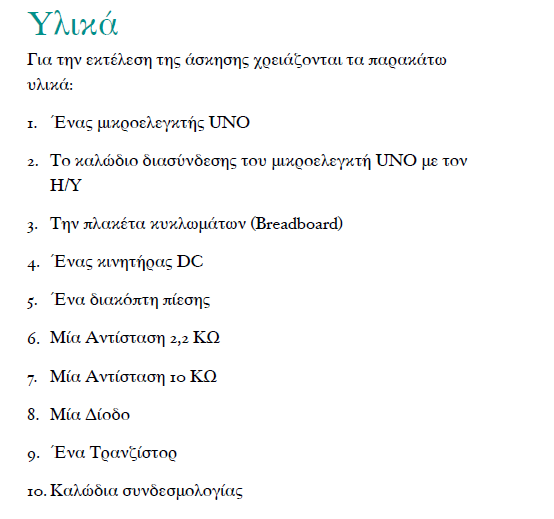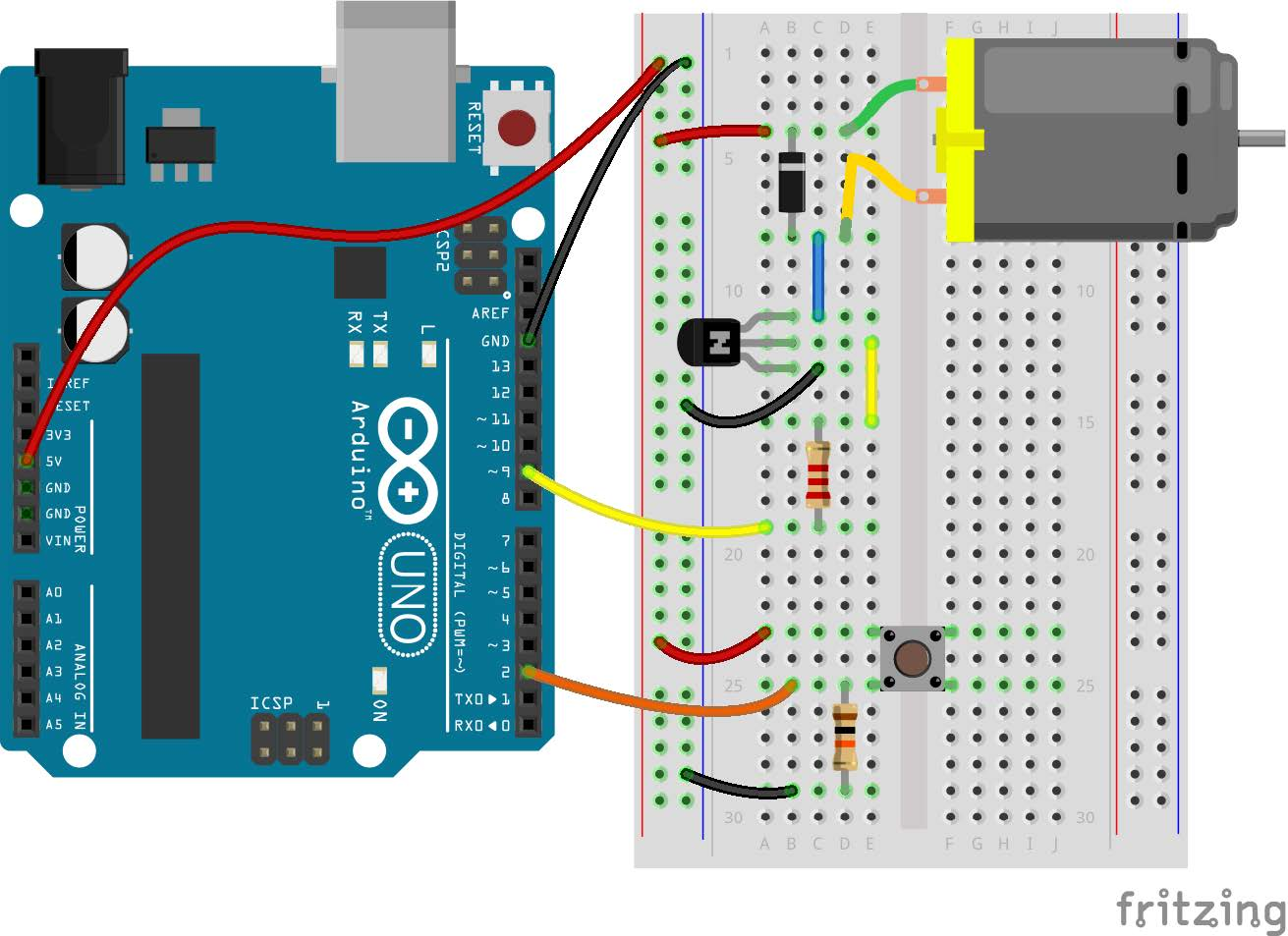tel. 24910 23755 / fax 24910 26620`/*`
`Make the motor spin while the pushbutton is`
`pressed and stop it when the pushbutton`
`is released.`
`*/`
`// constants won't change. They're used here to`
`// set pin numbers:`
`// in Pin 9 we will connect the motor control`
`// signal. Give it a name.`
`const int motorPin = 9;`
`// in Pin 2 we will connect the button control`
`// signal. Give it a name.`
`const int buttonPin = 2;`
`// variables will change:`
`// variable for reading the pushbutton status`
`int buttonState = 0;`
`// the setup routine runs once when you press`
`// reset:`
`void setup() {`
`  // initialize the motor pin as an output`
`  pinMode(motorPin, OUTPUT);`
`  // initialize the pushbutton pin as an input`
`  pinMode(buttonPin, INPUT);`
`}`
`// the loop routine runs over and over again`
`// forever:`
`void loop() {`
`  // read the state of the pushbutton value`
`  buttonState = digitalRead(buttonPin);`
`  // check if the pushbutton is pressed.`
`  // if it is, the buttonState is HIGH.`
`  if (buttonState == HIGH) {`
`   // spin motor`
`   digitalWrite(motorPin, HIGH);`
`  } else {`
`   // stop motor`
`   digitalWrite(motorPin, LOW);`
`  }`
`}`

Κατηγορίες: Arduino